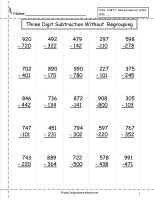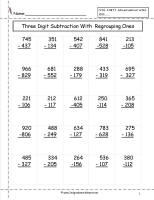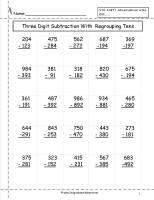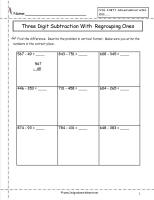﻿ Three Digit Subtraction Worksheets

# Three Digit Subtraction With and Without Regrouping Worksheets

## Three Digit Subtraction Without Regrouping, Three Digit Subtraction With Regrouping

###Three Digit Subtraction Without Regrouping Worksheets 2, 3, 4

Common Core State Standards: CCSS 2.NBT.7 Add and subtract within 1000……...
Three digit subtraction without regrouping.  25 problems.

###Three Digit Subtraction With Regrouping Worksheets 2, 3, 4

Common Core State Standards: CCSS 2.NBT.7 Add and subtract within 1000……...
Three digit subtraction with regrouping.  Students borrow from the tens place only. 25 problems.

###Three Digit Subtraction With Regrouping Worksheets 2, 3, 4

Common Core State Standards: CCSS 2.NBT.7 Add and subtract within 1000……...
Three digit subtraction with regrouping.  Students borrow from the hundreds place. 25 problems.

###Three Digit Subtraction Horizontal to Vertical Worksheet

Common Core State Standards: CCSS 2.NBT.7 Add and subtract within 1000……...
Three digit subtraction with regrouping.  Students rewrite problems from horizonal format to vertical format.